AP Calculus AB : Integrals

Example Questions

1 2 24 25 26 27 28 29 30 32 Next →

Example Question #43 : Trapezoidal Sums

Evaluate the integral using the trapezoidal sums method: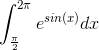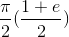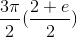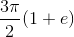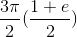Explanation:

To solve the integral, we will use the formula for the trapezoidal sums method: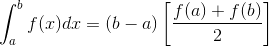Using the integral from the problem statement, we get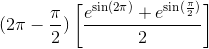Simplifying, we end up withExample Question #44 : Trapezoidal Sums

Evaluate using the trapezoidal approximation: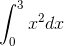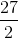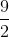Explanation:

The trapezoidal approximation of a definite integral is given by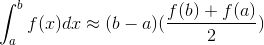For our integral, we get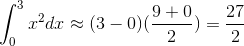Example Question #45 : Trapezoidal Sums

Use the method of trapezoidal sums to approximate the integral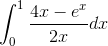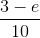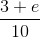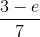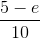Explanation:

To approximate the integral using trapezoidal sums, we use the following formula: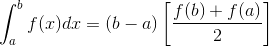Using the integral from the problem statement, we get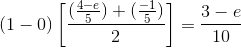Example Question #46 : Trapezoidal Sums

Using the method of trapezoidal sums, evaluate the following integral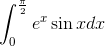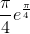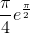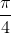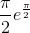Explanation:

To find the value of the integral, we use the following formula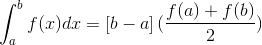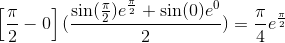Example Question #47 : Trapezoidal Sums

Using the method of trapezoidal sums, evaluate the following integral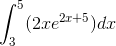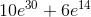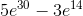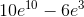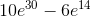Explanation:

To use the method of trapezoidal sums, we follow the definitionUsing the information from the problem statement, we get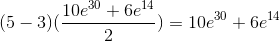Example Question #48 : Trapezoidal Sums

Use the trapezoidal approximation to solve the definite integral, and find the difference between it and the actual integral: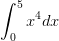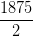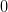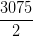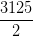Explanation:

The trapezoidal approximation to definite integrals is given by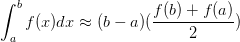Using this formula for our integral, we get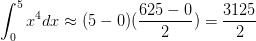Actually integrating, we get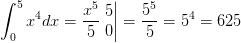The rule used for integration is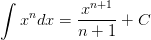The difference between the approximation and the actual answer is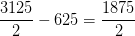Example Question #49 : Trapezoidal Sums

Approximate the value of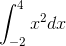using a trapezoidal sum with step size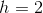. How far away is this approximation from the actual value of the integral above?

6

5

10

2

4

4

Explanation:

Trapezoidal sums are found by creating trapezoids whose left and right end points are on the specified function, and whose widths are the step size. We then sum up their areas by remembering that the area of a trapezoid is the base times the average of the heights.

Thus, the calculation of the trapezoidal sum for this example would be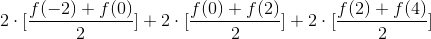A more simplified version would be given by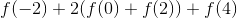Which evaluates to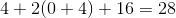The actual answer is found by evaluating the definite integral given, which would just be given by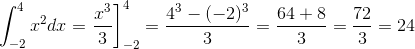The difference between the approximation and the the true answer is thus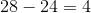1 2 24 25 26 27 28 29 30 32 Next →